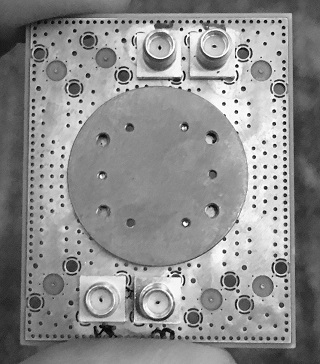# Quantum computing

A Quantum computer is a computer based on quantum mechanics. Quantum mechanics is a scientific theory that explains how tiny particles like atoms and electrons behave and interact with each other. Quantum mechanics deals with very small particles and operates on principles like probability and uncertainty.

A quantum computer does not use classical CPUs or GPUs, but a processor based on so-called qubits. A qubit (or quantum bit) is the basic unit of quantum information. Unlike classical bits, which can be either 0 or 1, qubits can exist in a superposition of states, representing multiple values simultaneously. This property enables quantum computers to perform certain tasks much faster than classical computers.The number of qubits in a quantum computer is not comparable to the number of transistors in a CPU. The idea behind a quantum computer is that instead of calculating all the possibilities of a problem, a quantum computer can determine all the solutions at once. A problem with 1 billion possibilities can be computed with 30 qubits at once.

But computing is not the right word. Traditional computers are deterministic and quantum computers are probabilistic. Deterministic means that the result is predetermined, and every time a calculation is performed, the answer will be the same. Probabilistic means that there is a high probability that the result is correct, but that each computation is an approximation that may produce a different result each time. Because of the uncertainty inherent in quantum mechanics by definition, the answer is always an approximation.

Qubits are also highly unstable - they must be cooled to near absolute zero to become superconducting, and they can only hold a stable position for a few milliseconds. This means that calculations have to be repeated many times to get a sufficiently reliable answer.

Quantum computers are still in the experimental stage. A few research centers and large companies like IBM are working on them. Given the complexity and cooling requirements, quantum computing capabilities will most likely be offered as a cloud service in the future.

Quantum computing can be used in medicine, for example, it could speed up drug discovery and help medical research by speeding up chemical reactions or protein folding simulations, something that will never be possible with classical computers because it would take thousands of years to calculate on a classical supercomputer.

Because of its properties, quantum computing could easily break current encryption systems. Therefore, cryptographers are working on post-quantum algorithms.

IBM has built the largest quantum computer yet, with 433 qubits. This figure shows the progression of the number of qubits in the largest quantum computers.This entry was posted on Donderdag 20 April 2023